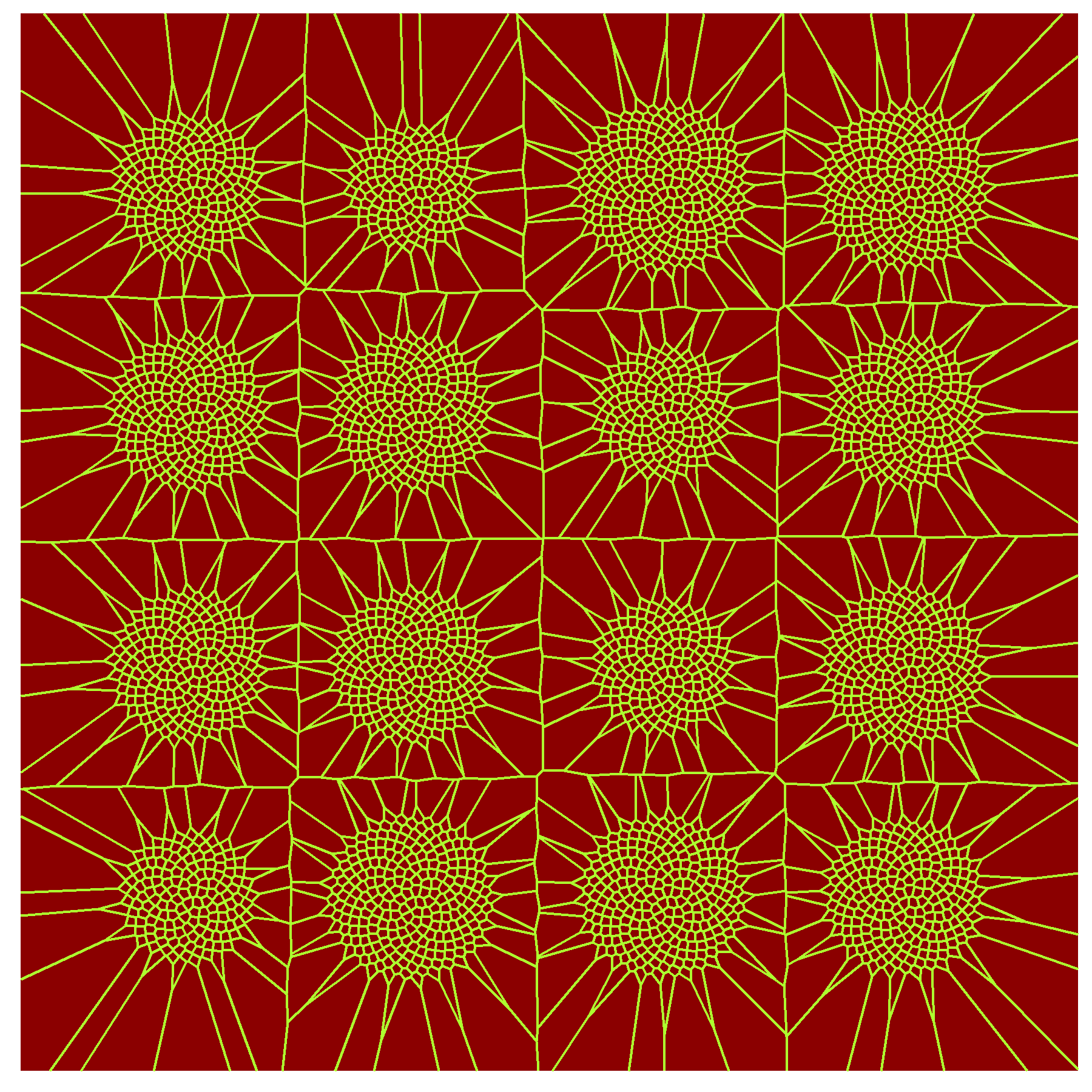# Sunflowers

The world is full of wonderful things, like sunflowers (Machanguito, my islander friend)

Sunflower seeds are arranged following a mathematical pattern where golden ratio plays a starring role. There are tons of web sites explaining this amazing fact. In general, the arrangement of leaves on a plant stem are ruled by spirals. This fact is called phyllotaxis, and I did this experiment about it some time ago. Voronoi tessellation originated by points arranged according the golden angle spiral give rise to this sunflowers:I know this drawing will like to my friend Machanguito because he loves sunflowers. He also loves dancing, chocolate cookies, music and swimming in the sea. Machanguito loves life, it is just that simple. He is also a big defender of renewable energy and writes down his thoughts on recycled papers. You can follow his adventures here.

This is the code:

```library(deldir)
library(ggplot2)
library(dplyr)
opt = theme(legend.position  = "none",
panel.background = element_rect(fill="red4"),
axis.ticks       = element_blank(),
panel.grid       = element_blank(),
axis.title       = element_blank(),
axis.text        = element_blank())
CreateSunFlower <- function(nob=500, dx=0, dy=0) {   data.frame(r=sqrt(1:nob), t=(1:nob)*(3-sqrt(5))*pi) %>%
mutate(x=r*cos(t)+dx, y=r*sin(t)+dy)
}
g=seq(from=0, by = 45, length.out = 4)
jitter(g, amount=2) %>%
expand.grid(jitter(g, amount=2)) %>%
apply(1, function(x) CreateSunFlower(nob=round(jitter(220, factor=15)), dx=x, dy=x)) %>%
do.call("rbind", .) %>% deldir() %>% .\$dirsgs -> sunflowers
ggplot(sunflowers) +
geom_segment(aes(x = x1, y = y1, xend = x2, yend = y2), color="greenyellow") +
scale_x_continuous(expand=c(0,0))+
scale_y_continuous(expand=c(0,0))+
opt
```